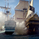2340 visitas
2340
This scalper uses elder's force index cross over to provide trade signal. Unlike the simple, ema cross over, forces index takes volume into consideration.
Besides it uses ATR to filter out bad signals in the sideway period, and give quality signals only when volatility comes.
The result is fewer false signals and better trade.
```study(title="yuthavithi volatility based force trade scalper", shorttitle="YUTHAVITHI Volatility based FORCE Scalper", overlay=true)
fast = input(3, minval= 1, title="Fast")
slow = input(20, minval = 1, title = "Slow")
atrFast = input(20, minval = 1, title = "ATR Fast")
atrSlow = input(50, minval = 1, title = "ATR Slow")

len = input(20, minval=1, title="Length")
multiplier = input(2, minval=1, title="multiplier")
src = input(close, title="Source")
bbMid = sma(src, len)
plot(bbMid, color=blue)

atrFastVal = atr(atrFast)
atrSlowVal = atr(atrSlow)
stdOut = stdev(close, len)
bbUpper = bbMid + stdOut * multiplier
bbLower = bbMid - stdOut * multiplier
plot(bbUpper, color = (atrFastVal > atrSlowVal ? red : silver))
plot(bbLower, color = (atrFastVal > atrSlowVal ? red : silver))

force = volume * (close -  nz(close))
xforce = cum(force)
xforceFast = ema(xforce, fast)
xforceSlow = ema(xforce, slow)

bearish = ((xforceFast < xforceSlow) and (atrFastVal > atrSlowVal)) and ((xforceFast > xforceSlow) or (atrFastVal < atrSlowVal)) and (close < open)
bullish = ((xforceFast > xforceSlow) and (atrFastVal > atrSlowVal)) and ((xforceFast < xforceSlow) or (atrFastVal < atrSlowVal)) and (close > open)

plotshape(bearish, color=red, style=shape.arrowdown, text="Sell", location=location.abovebar)
plotshape(bullish, color=green, style=shape.arrowup, text="Buy", location=location.belowbar)
```Great stuff youngyuth! I really like your BB Scalper too. I just added this script to my favorites. The 'BUY' & 'SELL' label and arrow is not showing up. Any suggestions? Also, I have an idea for a script using regression trend bands. Would you be interested in looking at it and perhaps writing a script? Should be pretty easy and straight forward. Thanks.
Responder